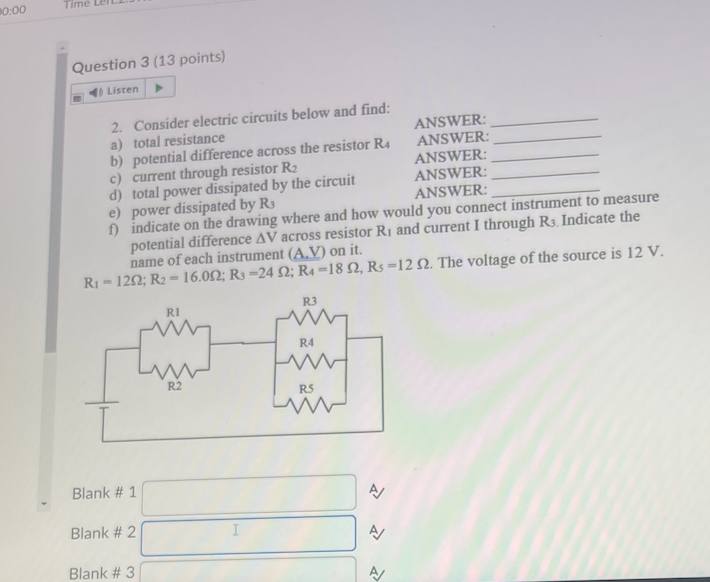# Question Please explain 2. Consider electric circuits below and find: a) total resistance ANSWER: b) potential difference across the resistor R4​ ANSWER: c) current through resistor R2​ ANSWER: d) total power dissipated by the circuit ANSWER: e) power dissipated by R3​ ANSWER: f) indicate on the drawing where and how would you connect instrument to measure potential difference ΔV across resistor R1​ and current I through R3​. Indicate the name of each instrument (A,V) on it. R1​=12Ω;R2​=16.0Ω;R3​=24Ω;R4​=18Ω,R5​=12Ω. The voltage of the source is 12 V.